# Mean Absolute Deviation Lesson Plan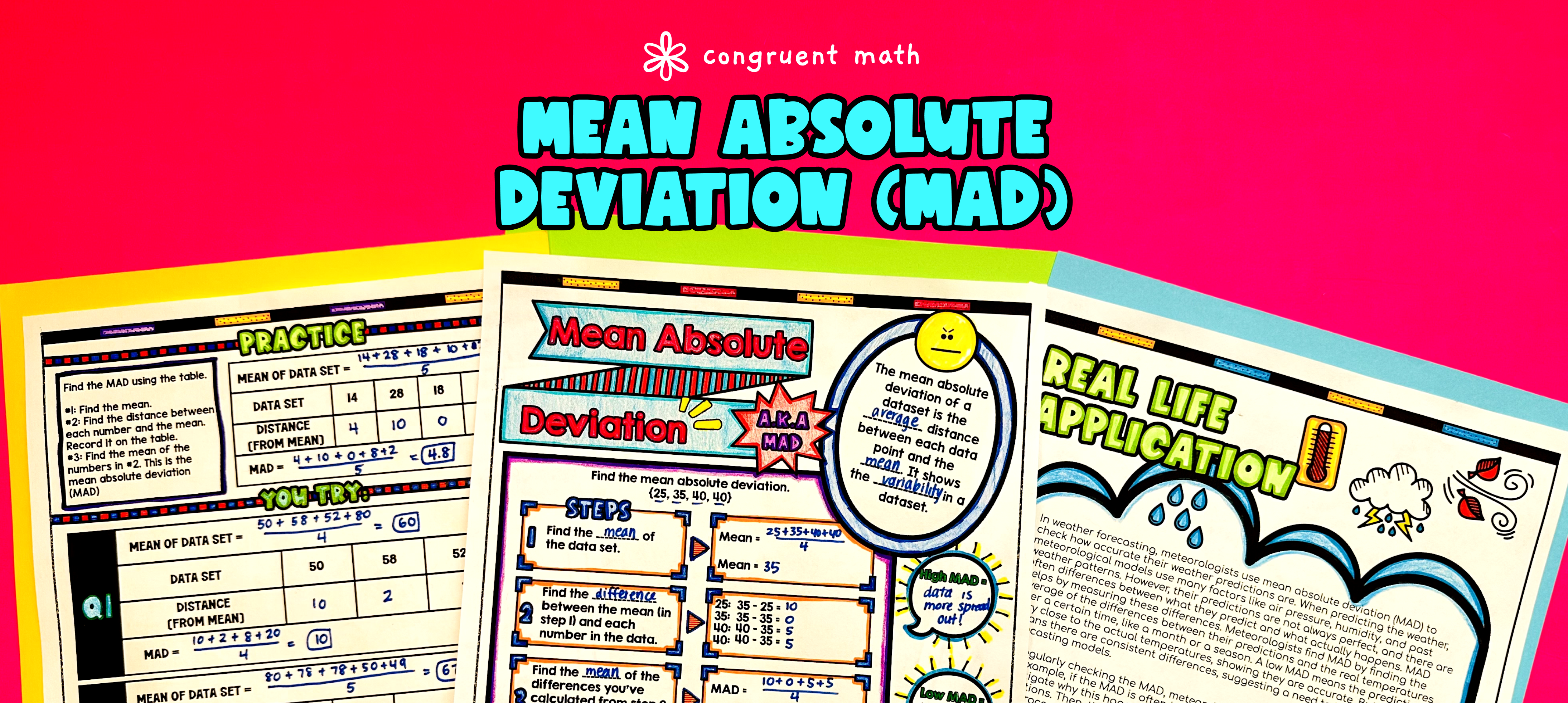Pin This

## Overview

Ever wondered how to teach mean absolute deviation in an engaging way to your sixth-grade students?

In this lesson plan, students will learn about mean absolute deviations (MAD) and their real-life applications. Through artistic, interactive guided notes, check for understanding questions, a doodle activity, a color by number activity, and a maze worksheet, students will gain a comprehensive understanding of this concept.

The lesson culminates with a real-life application activity where students will read and write about real-life uses of MAD. This activity helps students connect the concept of MAD to its practical applications in the world.

## Get the Lesson Materials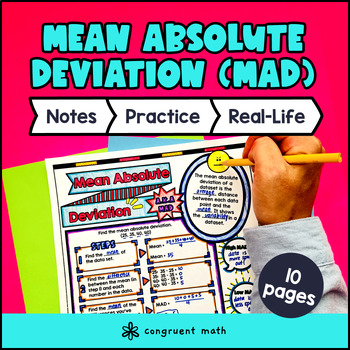\$4.25

## Learning Objectives

After this lesson, students will be able to:

• Define mean absolute deviation (MAD) as a measure of variability in a set of data

• Calculate the mean absolute deviation of a given data set

• Explain real-life applications of mean absolute deviation in various contexts

## Prerequisites

Before this lesson, students should be familiar with:

• Knowledge of the mean and how to calculate it

• Basic math skills, including addition, subtraction, multiplication, and division of positive integers and decimals

## Key Vocabulary

• Statistics

• Measure of Variability

## Procedure

### Introduction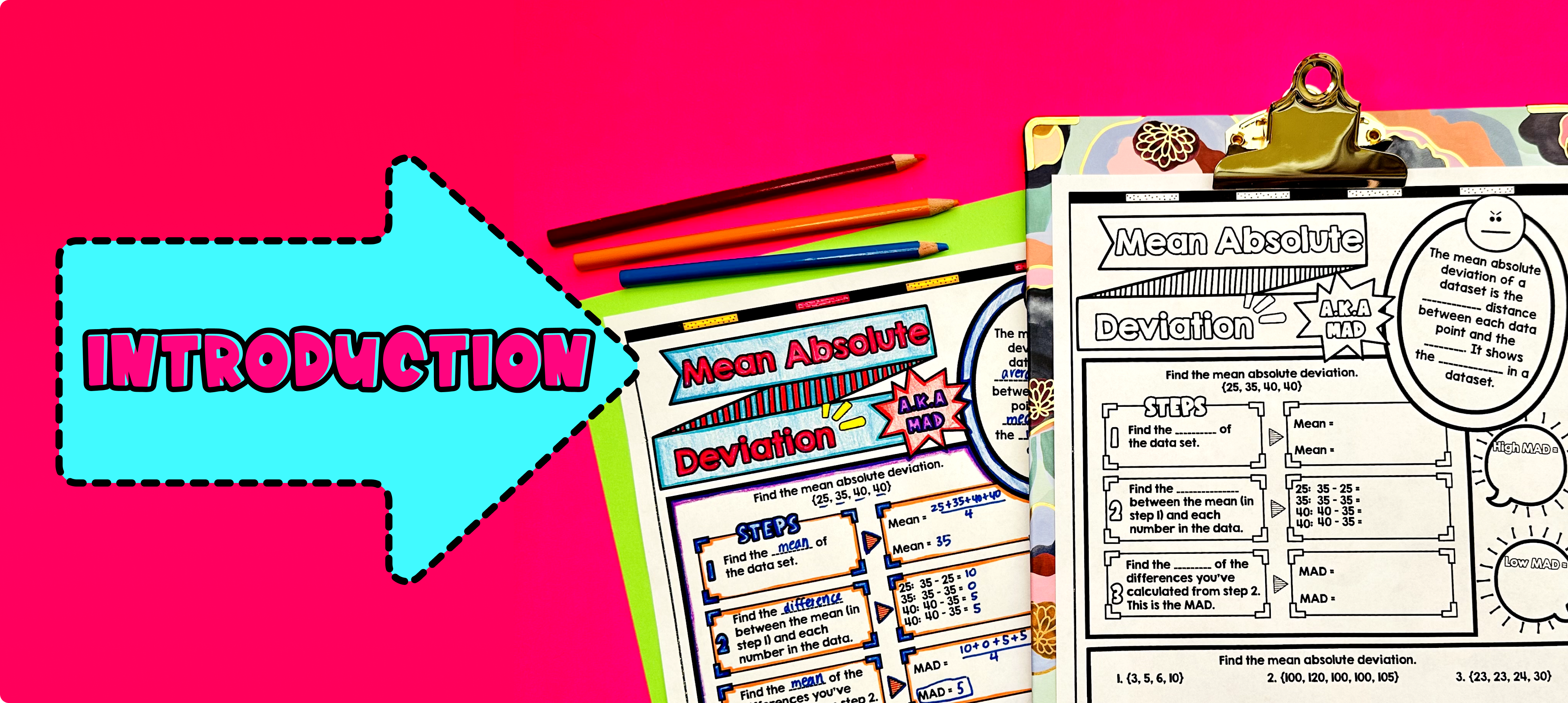Pin This

As a hook, ask students why understanding variability in data is important in real life. You can provide examples like comparing the variability of temperatures in different cities, or comparing the variability in test scores of different classrooms. Refer to the last page of the guided notes as well as the FAQs below for ideas.

Use the first page of the guided notes to introduce mean absolute deviation (MAD) and its significance as a measure of variability. Explain that MAD measures how spread out the data values are from the mean. Walk through the key points of the topic of the guided notes to teach, including the 3 steps to calculate MAD.

Based on student responses, reteach concepts that students need extra help with. If your class has a wide range of proficiency levels, you can pull out students for reteaching, and have more advanced students begin work on the practice exercises.

### Practice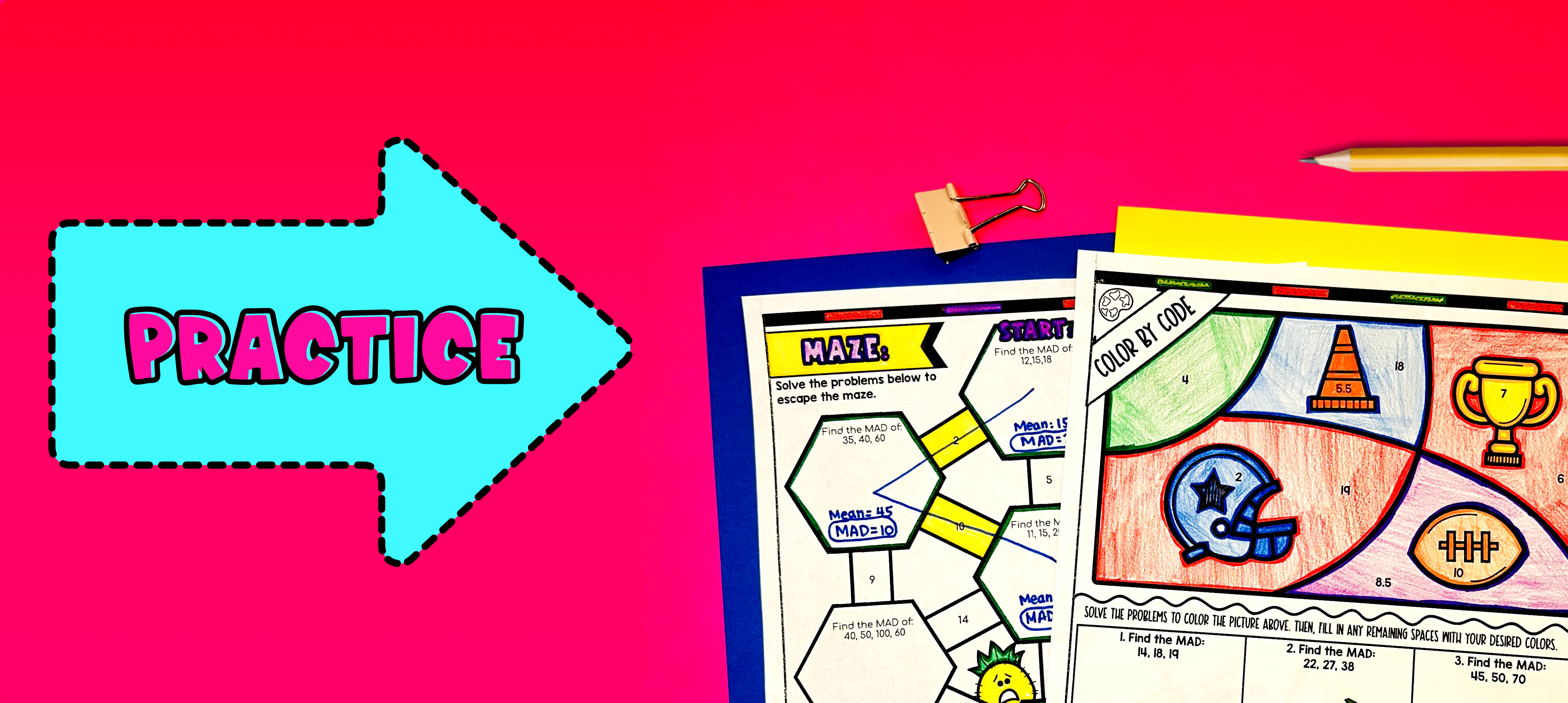Pin This

Have students practice calculating mean absolute deviation using the second page of the guided notes. The practice problems include tables which break down the problem into 3 steps. Step 1 is to calculate the mean of the data set. Step 2 is to calculate the difference between the data value and the mean. Lastly, step 3 is to calculate the MAD by finding the mean of the numbers in Step 2.

Fast finishers can then move on to the maze activity (page 3) and color by number activity (page 4) for additional practice. You can assign the practice worksheet as homework for the remainder of the class.

### Real-Life Application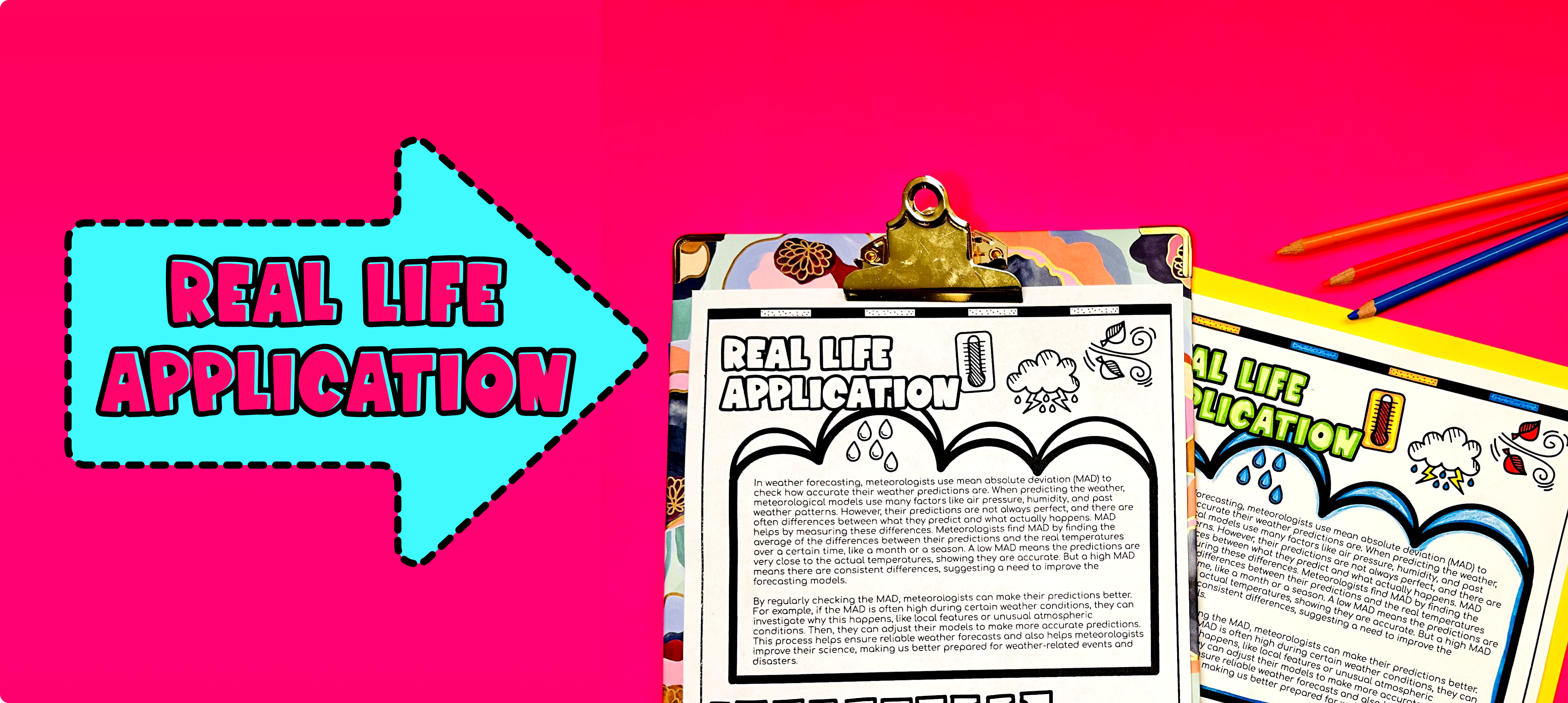Pin This

Bring the class back together, and introduce the concept of real-life applications of mean absolute deviation. Discuss how mean absolute deviation is a measure of how spread out the data is from the mean. Explain that mean absolute deviation is used in various real-life situations to understand the variability or dispersion of a data set.

Use the last page of the guided notes for students to read about a detailed example of MAD in weather forecasting and analysis. For example, you can discuss how mean absolute deviation is used in analyzing weather data. Explain how meteorologists use mean absolute deviation to measure the variability in temperature or rainfall over a period of time. A higher mean absolute deviation indicates a greater variability in weather conditions, while a lower mean absolute deviation suggests more consistent weather patterns.

You can also mention other real-life applications of mean absolute deviation, such as in analyzing test scores.

Refer to the FAQs section of the lesson plan for more ideas on how to engage students in exploring and discussing real-life applications of mean absolute deviation. Encourage students to think of other examples on their own and share with the class.

## Extensions

A fun, no-prep way to practice mean absolute deviation is Doodle Math — they’re a fresh take on color by number or color by code. It includes multiple levels of practice, perfect for a review day or sub plan.

Here are some activities to try:

If you’re looking for digital practice for simple interest, try my Pixel Art activities in Google Sheets. Every answer is automatically checked, and correct answers unlock parts of a mystery picture. It’s incredibly fun, and a powerful tool for differentiation.

Here are some activities to explore:

### Codebreaker (Video & Print Activity):

A fun way to wrap this lesson with your students is with one of my math codebreaker video and print activities. Students watch a video that immerses them as detectives, having to solve math problems to find the secret code. If students successfully solve the math problems and find the code, you would play the mission success video to end the activity.

Here are some activities to explore:

## Want more ideas and freebies?

Get my free resource library with digital & print activities—plus tips over email.